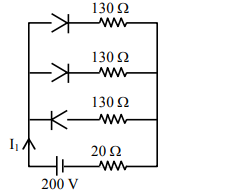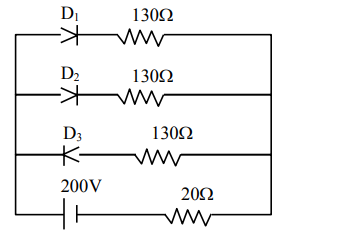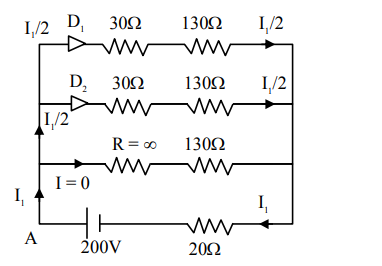# In the given figure, each diode has a forward bias resistanceQuestion:

In the given figure, each diode has a forward bias resistance of $30 \Omega$ and infinite resistance in reverse bias. The current $I_{1}$ will be :1.  3.75 A

2. 2.35 A

3. 2 A

4. 2.73 A

Correct Option: , 3

Solution:As per diagram, Diode D1 & D2 are in forward bias i.e. R = 30: whereas diode D3 is in reverse bias i.e. R = infinite

$\Rightarrow$ Equivalent circuit will be

Applying KVL starting from point A$-\left(\frac{I_{1}}{2}\right) \times 30-\left(\frac{I_{1}}{2}\right) \times 130-I_{1} \times 20+200=0$

$\Rightarrow-100 \mathrm{I}_{1}+200=0$

$\mathrm{I}_{1}=2$